# Jump Statements in CChallenge Inside! : Find out where you stand! Try quiz, solve problems & win rewards!

## Overview

Jump statements in C are used to interrupt the flow of the program or escape a particular section of the program. There are many more operations they can perform within the loops, switch statements, and functions. There are four types of jump statements. The working and execution of the four types of jump statements can be seen in this article.

## Scope

• This article covers all the types of jump statements in C language.
• The article is example-oriented, along with the flow charts, syntax, and C program of each jump statement.
• The article covers the advantages and disadvantages of jump statements along with FAQs.

## Introduction

Jump Statements interrupt the normal flow of the program while execution and jump when it gets satisfied given specific conditions. The main uses of jump statements are to exit the loops like for, while, do-while also switch case and executes the given or next block of the code, skip the iterations of the loop, change the control flow to specific location, etc.

## Types of Jump Statement in C

There are 4 types of Jump statements in C language.

1. Break Statement
2. Continue Statement
3. Goto Statement
4. Return Statement.

## Break Statement in C

Break statement exits the loops like for, while, do-while immediately, brings it out of the loop, and starts executing the next block. It also terminates the switch statement.

If we use the break statement in the nested loop, then first, the break statement inside the inner loop will break the inner loop. Then the outer loop will execute as it is, which means the outer loop remains unaffected by the break statement inside the inner loop.

The diagram below will give you more clarity on how the break statement works.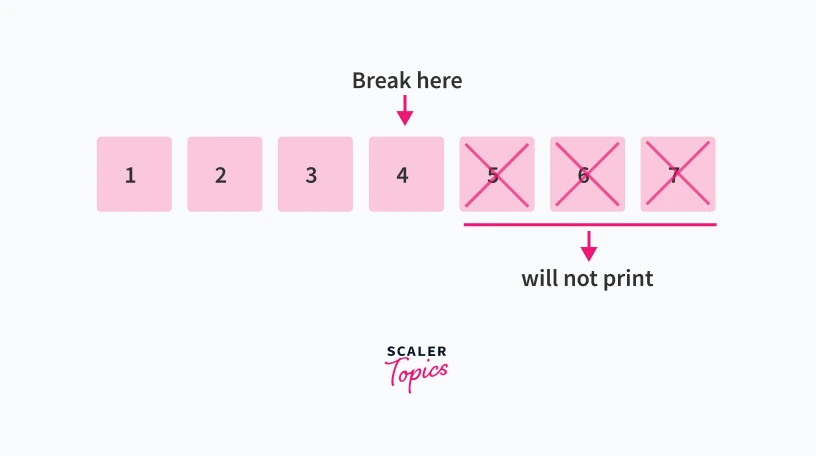### Syntax of Break Statement

The break statement's syntax is simply to use the break keyword.

Syntax:

``````//specific condition
break;
``````

### Flowchart of Break Statement

Flowchart of the break statement in the loop:

The break statement is written inside the loop.

• First, the execution of the loop starts.
• If the condition for the loop is true, then the body of the loop executes; otherwise, the loop terminates.
• If the body of the loop executes, the break condition written inside the body is checked. If it is true, it immediately terminates the loop; otherwise, the loop keeps executing until it meets the break condition or the loop condition becomes false.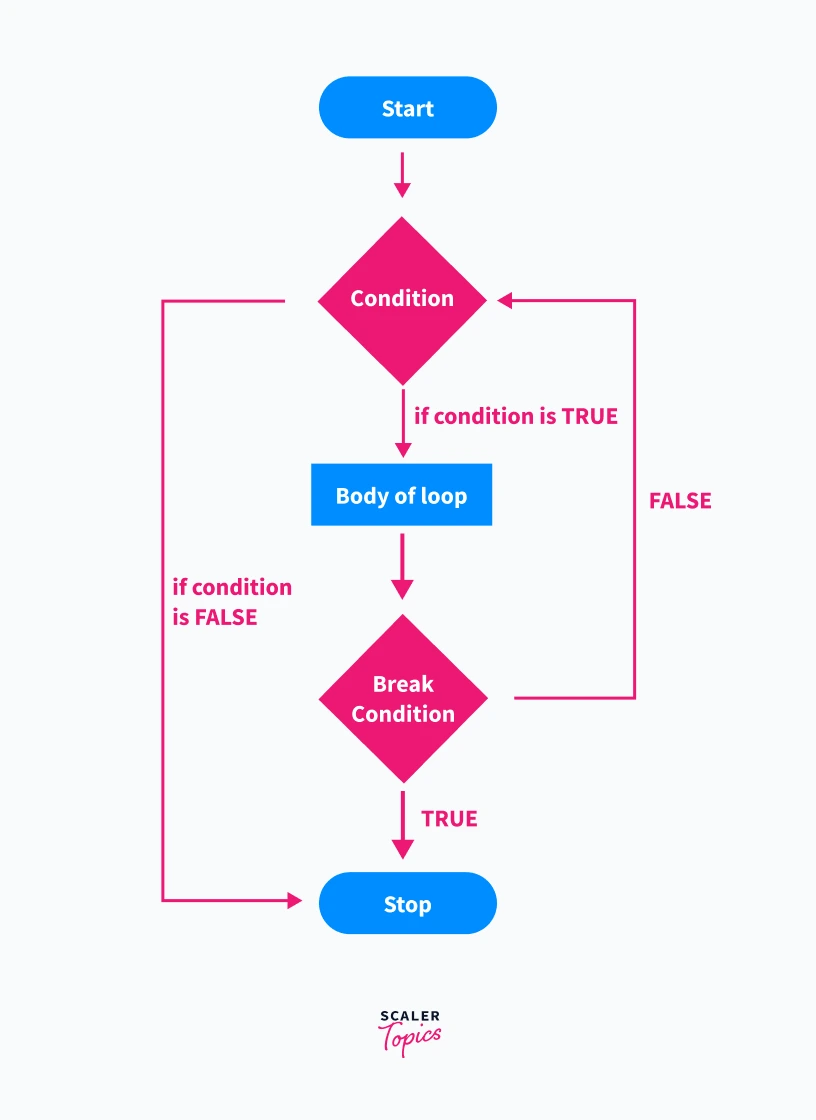Flowchart of the break statement in switch:

The switch case consists of a conditional statement. According to that conditional statement, it has cases. The break statement is present inside every case.

• If the condition inside the case for the conditional statement is true, then it executes the code inside that case.
• After that, it stops the execution of the whole switch block due to encountering a break statement and breaking out of it.

Note: If we don't use break-in cases, all cases following the satisfied case will execute.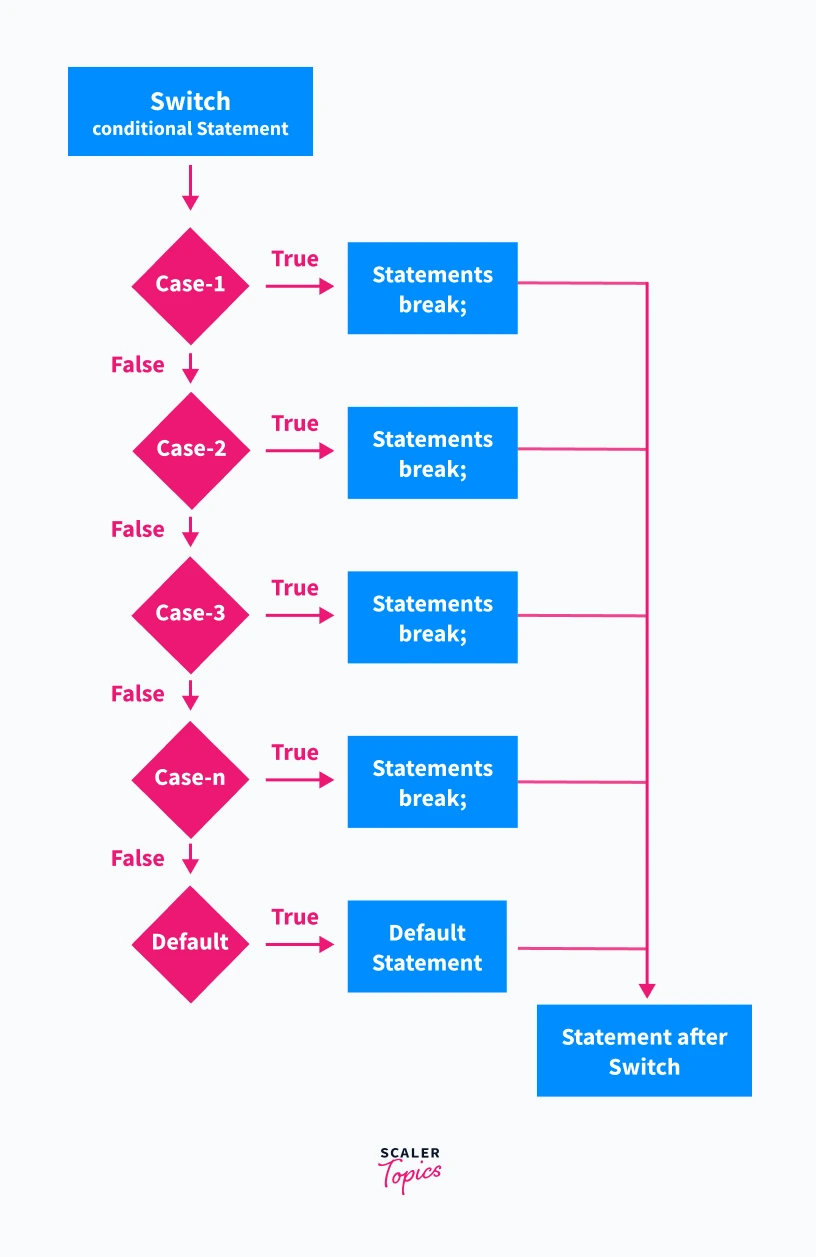So, to better understand, let's go for a C Program.

### Example: How does Break Statement in C Works?

In a while loop:

The while loop repeatedly executes until the condition inside is true, so the condition in the program is the loop will execute until the value of a is greater than or equal to 10. But inside the while loop, there is a condition for the break statement: if a equals 3, then break. So the code prints the value of a as 1 and 2, and when it encounters 3, it breaks(terminates the while loop) and executes the print statement outside the while loop.

Code:

``````#include<stdio.h>

void main() {

int a = 1; //initialize value to a

while (a <= 10) // run loop unless the value is 10
{
if (a == 3) // if the value is 3
break; //break the loop

printf("Print=%d \n", a);
a++;
}

printf("Outside loop"); //print statement outside the loop
}
``````

Output:

``````  Print=1
Print=2
Outside loop
``````

In switch statement:

As the switch statement consists of conditional statements and cases, here we have the conditional statement switch(a), and we have cases where we have different values of a.

The program will take the values of a from the user, and if any value entered by a user matches the value of a inside any case, then the code inside that case will completely execute and will break(terminate the switch statement) to end. If the value of a doesn't match any value of a inside the cases, then the default case will execute.

Code:

``````#include<stdio.h>

void main() {

int a = 0; //initialize a=0
printf("Enter a number: ");
scanf("%d", & a); //

switch (a) {

case 1: // if a=1 execute case and break
printf("Outstanding\n");
break;

case 2: // if a=2 execute case and break
printf("Good\n");
break;

case 3: // if a=3 execute case and break
printf("Average\n");
break;

default: // if value of a doesn't matches with case

}
printf("Outside Switch"); //outside the switch statement
}
``````

Output:

``````Enter a number: 2
Good
Outside Switch
``````

Example: Use of Break Statement in Nested Loop.

In the below code, the inner loop is programmed to perform for 8 iterations, but as soon as the value of j becomes greater than 4 it breaks the inner loop and stops execution and the execution of the outer loop remains unaffected.

Code:

`````` #include <stdio.h>

int main() {

for (int i = 0; i < 5; i++) {
for (int j = 1; j <= 8; j++) {

if (j > 4) //inner loop break condition
break;
else
printf("1");
}
printf("\n");
}

return 0;
}
``````

Output:

``````1111
1111
1111
1111
1111
``````

Example: Checking if the entered number is prime or not using break statement

In the below code, if we find out that the modulo of the number a with a for loop i from 2 to a/2 is zero, then we don't need to check further as we know the number is not prime, so we use break statement to break out from the loop.

Code:

``````#include<stdio.h>

int main()
{
int a, i;
printf("Enter a positive number:");
scanf("%d",&a);

for(i = 2; i <= a/2; i++) //for loop
{
if(a % i == 0) //break condition
{
break; //break statement
}
}

if (i > a/2) //after breaking the loop if i is greater than a/2
printf("\n %d is Prime Number \n",a);
else
printf("\n %d is Not a Prime Number \n",a);
return 0;
}
``````

Output:

``````Enter a positive number:5

5 is Not a Prime Number
``````

## Continue Statement in C

Continue in jump statement in C skips the specific iteration in the loop. It is similar to the break statement, but instead of terminating the whole loop, it skips the current iteration and continues from the next iteration in the same loop. It brings the control of a program to the beginning of the loop.

Using the continue statement in the nested loop skips the inner loop's iteration only and doesn't affect the outer loop.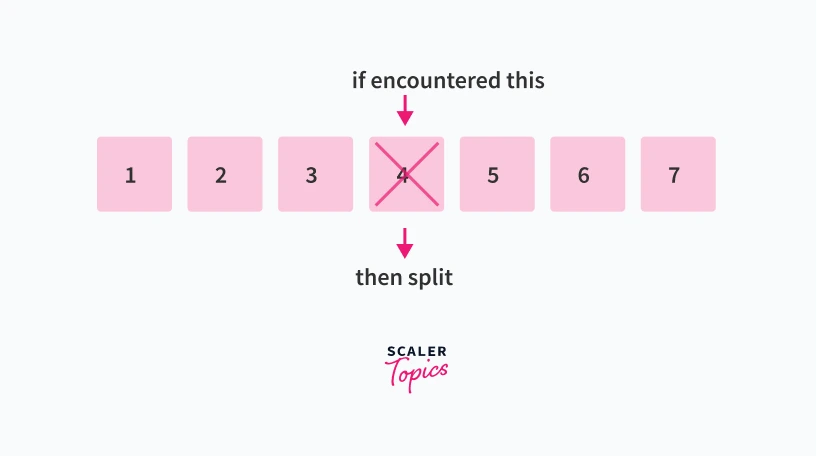### Syntax of continue Statement

The syntax for the continue statement is simple continue. It is used below the specified continue condition in the loop.

Syntax:

``````continue;
``````

### Flow Chart of Continue Statement in C

In this flowchart:

• The loop starts, and at first, it checks if the condition for the loop is true or not.
• If it is not true, the loop immediately terminates.
• If the loop condition is true, it checks the condition for the continue statement.
• If the condition for continue is false, then it allows for executing the body of the loop.
• Otherwise, if the condition for continue is true, it skips the current iteration of the loop and starts with the next iteration.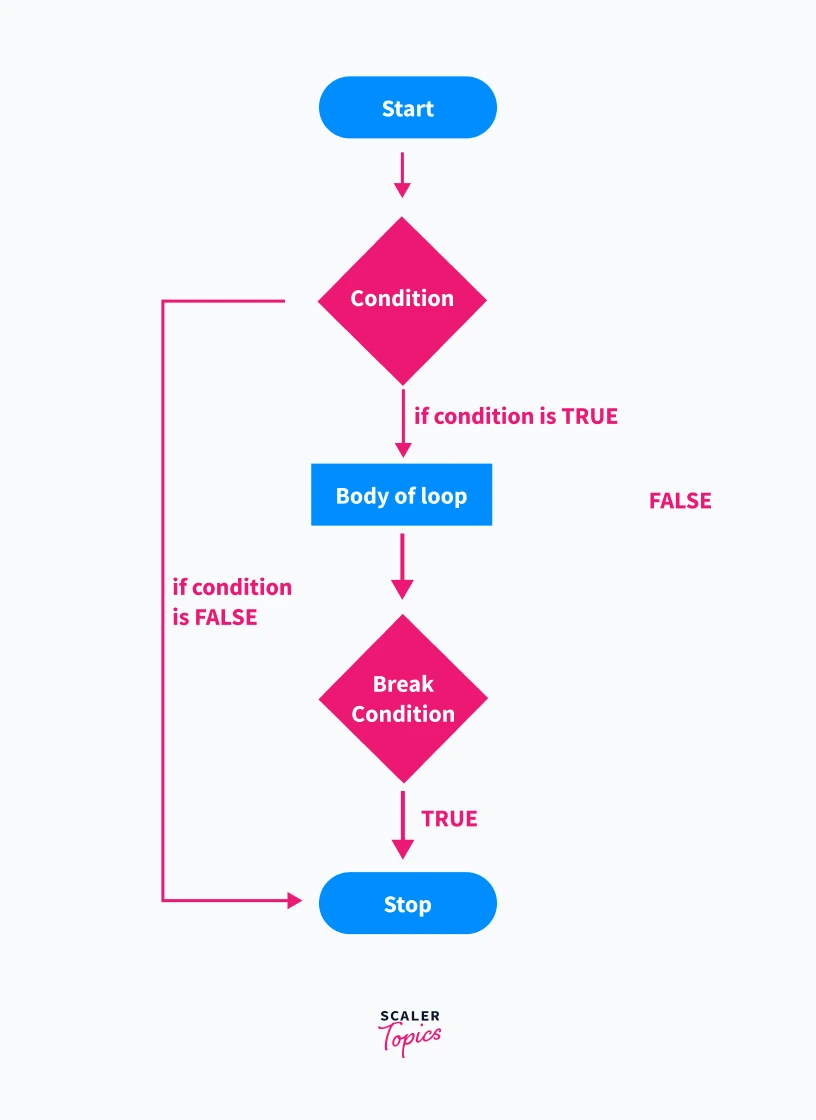### Uses of Continue Function in C

• If you want a sequence to be executed but exclude a few iterations within the sequence, then continue can be used to skip those iterations we don't need.

### Example: How does Continue Statement in C Works?

In the below program, when the continue condition gets true for the iteration i=3 in the loop, then that iteration is getting skips, and the control goes to the update expression of for loop where i becomes 4, and the next iteration starts.

Code:

``````#include<stdio.h>

int main()
{
int i;
for ( i = 1; i <= 7; i++ )
{
if( i == 3) //continue condition
{
continue; //continue statement
}

printf("Value = %d \n",i);
}
}

``````

Output:

``````Value = 1
Value = 2
Value = 4
Value = 5
Value = 6
Value = 7
``````

### Example: C continue statement in nested loops

Rolling 2 dices and count all cases where both dice shows different number:

In the program, the continue statement is used in a nested loop. The outer for loop is for dice1, and the inner for loop is for dice 2. Both the dices are rolling when the program executes since it is a nested loop. If the number of dice1 equals a number of dice 2 then it skips the current iteration, and program control goes to the next iteration of the inner loop.

We know that dice will show the same number 6 times, and in total, there are 36 iterations so that the Output will be 30.

Code:

``````#include<stdio.h>
void main()
{
int d1, d2, count = 0;
for( d1 = 1; d1 <= 6; d1++)
{
for( d2 = 1; d2 <= 6 ; d2++)
{
if (d1==d2) //if dice1 value equals dice 2 value
{
continue;
}

count = count + 1;
}
}
printf("The dices show different numbers %d times",count);
}
``````

Output:

``````The dices show different numbers 30 times
``````

## Goto Statement in C

Goto statement is somewhat similar to the continue statement in the jump statement in C, but the continue statement can only be used in loops, whereas Goto can be used anywhere in the program, but what the continue statement does it skips the current iteration of the loop and goes to the next iteration, but in the goto statement we can specify where the program control should go after skipping.

The concept of label is used in this statement to tell the program control where to go. The jump in the program that goto take is within the same function.

Below is the diagram; the label is XYZ, and it will be more clear when we'll see the syntax.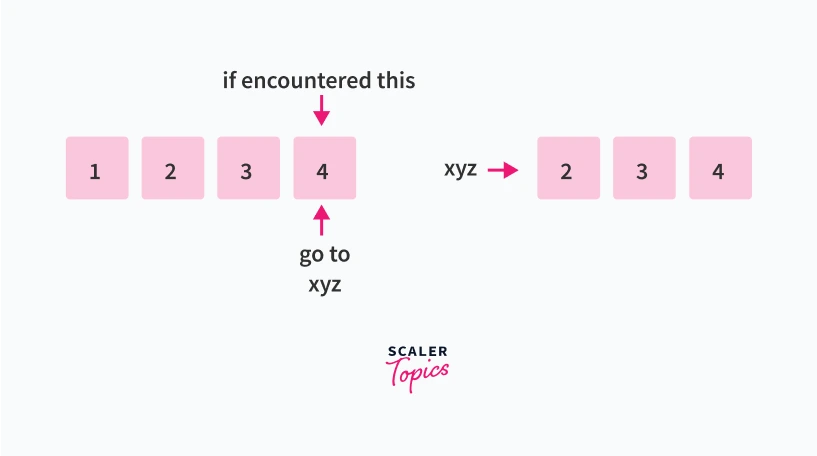### Syntax of goto Statement

Syntax:

``````goto label;
.
.
label:
--code--
--code--
``````

### Flow Chart of Goto Statement

Here statement 2 is unreachable for the program control as goto is written in statement 1, and label 3 is the label for statement 1.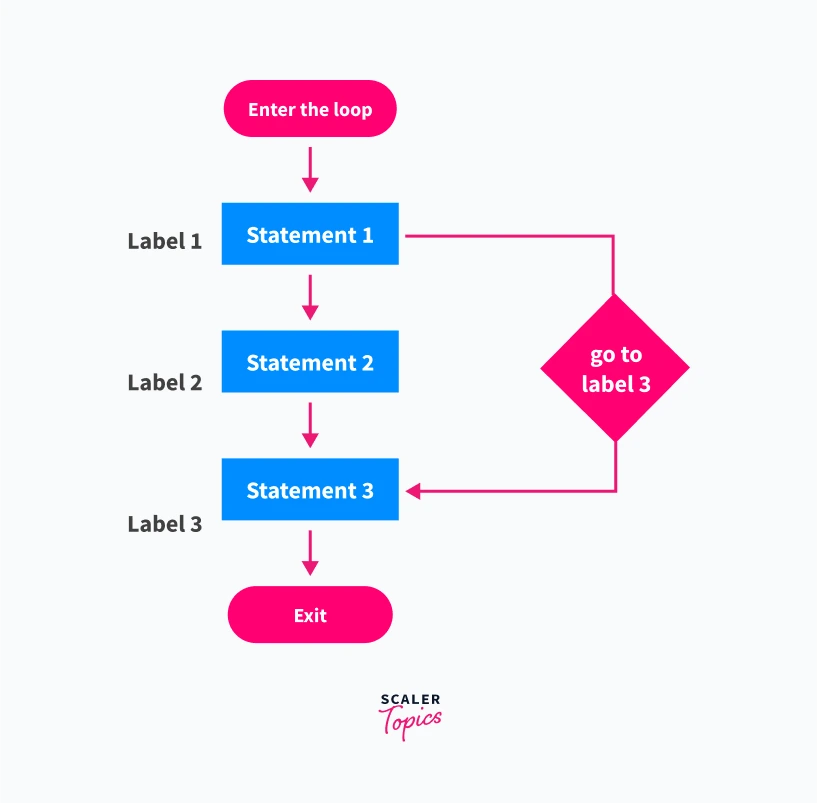It will be more clear with an example.

### Example: How does Goto Statement in C Works?

Let's take the example of odd and even numbers.

Check odd or even using goto:

In the program, the if statement has a logic of an even number. When it is satisfied, it will go to the Even label; otherwise, Odd Label.

Code:

``````#include<stdio.h>

int main() {

int a;
printf("\nEnter a Positive int:");
scanf("%d", & a);

if (a % 2 == 0) //logic of even no
goto Even; //goto statement 1
else
goto Odd; //goto statement 2

Even: // label 1
printf("Number is Even\n");
exit(0);

Odd: //label2
printf("Number is Odd\n");

return 0;
}
``````

Output:

``````Enter a Positive int:4
Number is Even
``````

### Example: Print counting 1 to 10 using goto function, without using any loop

In this program, the label has code to increment and print the value of an until it is greater than or equal to 10. The program will print the values until the label if the condition inside the label is true; otherwise, it will terminate the program.

Code:

``````#include<stdio.h>

int main() {

int a = 1; //initialised a=1

label: //label code
printf("%d\n", a);

a++;

if (a <= 10)
goto label; //goto statement

return 0;
}
``````

Output:

``````1
2
3
4
5
6
7
8
9
10
``````

### Example: Area of Square Using goto Statement

In the below program, the logic of the Area of the square is written inside the Square label, and the s, i.e., The side of a square, is taken from the user as input. If the value of s is greater than 0 then the program control will shift to the Square label, and if not, it will go to the next label, i.e., error, and exit the program.

Code:

``````#include<stdio.h>

int main() {

float a, s;
printf("\nEnter a side of square:");
scanf("%f", & a);

if (a > 0)
goto Square; //goto statement
else
goto error;

Square: // label
s = a * a;
printf("The Area of the square  is %f\n", s);
exit(0);
error: //label
printf("Enter a proper value!!");

return 0;
}
``````

Output:

``````Enter a side of square:5
The Area of the square  is 25.000000
``````

### Reasons to avoid goto statements

• If you are using goto, it is hard for the other person to trace the flow of the program and understand it.
• The program becomes hard to modify because it references the different labels, so rewriting is the only solution.
• The disadvantage of goto is that it could only be used inside the same function.

### Should you use goto?

• If you are planning to write a complex code for any purpose, you should avoid goto statements because debugging will make it more complex.
• If you want to understand that program and modify it, it will be very confusing.
• If you write goto for a minor program, it is fine, but using them for bigger ones is not suggested.

## Return Statement in C

The return statement is a type of jump statement in C which is used in a function to end it or terminate it immediately with or without value and returns the flow of program execution to the start from where it is called.

The function declared with void type does not return any value.

### Syntax of Return Statement

Syntax:

``````return expression;
or
return;
``````

### Flow Chart of Return Statement

In this flowchart, first, we call another function from the current function, and when that called function encounters a return statement, the flow goes back to the previous function, which we called another function.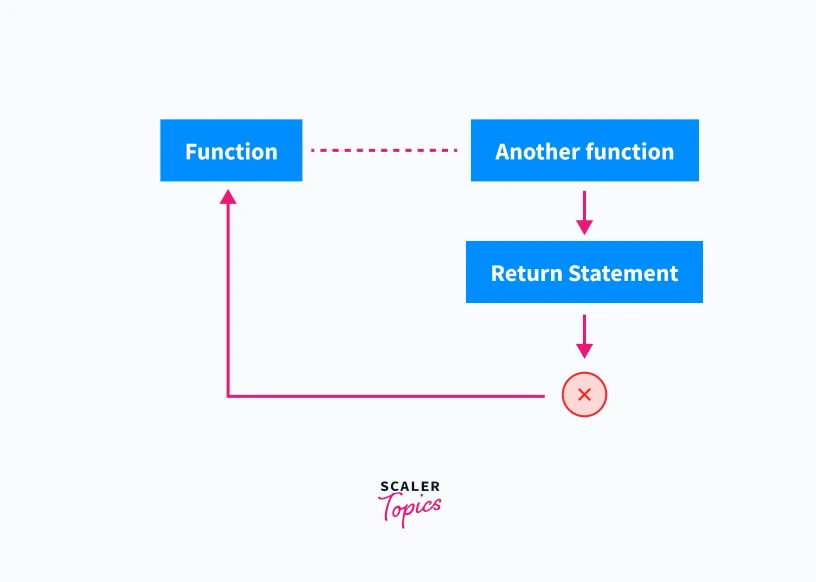### How does the return statement works in C?

The return statement is used in a function to return a value to the calling function. The return statement can be written anywhere and often inside the function definition. Still, after the execution of the first return statement, all the other return statements will be terminated and will be of no use.

The reason why we have the two syntaxes for the return statement is that the return statement can be used in two ways.

• First is that you can use it to terminate the execution of the function when the function is not returning any value.
``````return;
``````
• Second, it is to return a value with function calls, and here, the datatype of expression should be the same as the datatype of function.
``````return expression;
``````

Here the expression can be any variable, number, or expression. All the return examples given below are valid in C.

``````return x;
return 0;
return x++;
return(x+y);
return(3+2);
``````

But remember, the return statement always returns only one value. The return example given below is valid, but it will only return the rightmost value, i.e., c, because we have used a comma operator.

``````return a,b,c;
``````

Keeping this in mind, let's see an example to understand the return statement.

### Example: Program to understand the return statement

The program below is to calculate the sum of digits 1 to 10. Here first the int sum() block will execute where the logic is written to calculate the digits from 1 to 10 and after calculation, the return statement returns the value of a. Now the program control goes to the main function where the value of a is assigned coming from the sum(); function, and after printing the value of a, the return statement is used to terminate the main function.

Code:

``````#include<stdio.h>

int sum()
{
int a = 0;
int num;

for( num = 0; num <= 10 ; num++) {
a += num;
}

return a;
}

void  main()
{
int a = sum();
printf("Sum of digits from 1 to 10=%d\n",a);

return;
}
``````

Output:

``````Sum of digits from 1 to 10=55
``````

### Example: Using the return statement in void functions

In the code below, the return statement doesn't return anything, and we can see "level2" is not printed as the main function terminates after the return.

Code:

``````#include<stdio.h>
void main()
{
printf("level1\n");
return;
printf("level2\n");
}
``````

Output:

``````level1
``````

### Examples: Not using a return statement in void return type function

If the return statement inside the void returns any value, it can give errors. As seen in the code, the void Hello() method returns the value 10, whereas it is giving errors in the Output.

Code:

``````#include <stdio.h>
// void method
void Hello()
{
printf("Hello Scaler!");
return 10;// void returning value
}

//main method
int main()
{
// Calling Hello()
Hello();
return 0;
}
``````

Output:

``````main.c:12:6: note: declared here
12 | void Hello()
|      ^~~~~
Hello Scaler!
``````

• You can control the program flow or alter the follow of the program.
• Skipping the unnecessary code can be done using jump statements.
• You can decide when to break out of the loop using break statements.
• You get some sort of flexibility with your code using jump statements.

• Readability of the code is disturbed because there are jumps from one part of the code to another part.
• Debugging becomes a little difficult.
• Modification of the code becomes difficult.

## FAQs

1. What is the use of jump statements in C?

Jump Statements interrupt the normal flow of the program while execution and jump when it gets satisfied given specific conditions. It generally exits the loops and executes the given or next block of the code.

2. Why do we need Jump Function?

In between the loop, when we need to go to some other part of the code, jump statements shift the program control by breaking the loop, or if you need to skip the iteration in between the loop, the jump function does that.

It consists of the return statement by which you can return the values to the functions.

3. What is the main purpose of the goto function in C?

In the goto statement, we can specify where the program control should go in the current function by using the labels; you just need to specify the label name under which we want to shift the program control to.

4. Why should we avoid the goto statement in C?

If you are using goto, it is hard for the other person to trace the flow of the program and understand it. The program becomes hard to modify because it references the different labels, so rewriting is the only solution.

5. What is the rule of the continue function?

Continue statement skips the specific iteration in the loop and brings the program's control to the beginning of the loop. We could only use continue in loops.

6. Where do we use the continue function?

If you want a sequence to be executed but exclude a few iterations within the sequence, then the continue statement can be used.

For example, if you want to print 1 to 10 integers using the for loop and skip integer 5 from printing, then you can specify the condition and write the continue statement under it.

## Conclusion

• The jump statements in C are used in loops like for, while, do-while and break statement also covers switch statement, they simply manipulate the flow of the program control, using them we can achieve many things.
• The jump statements can be alternative to some loops like for loop(refer to example to print 1 to 10 numbers in goto statements).
• There are 4 types of jump statements break, continue, goto, and return.
• The jump statement has good and bad sides that we have seen with the goto statement.
Free Courses by top Scaler instructors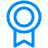Certificates
C Tutorial
This program includes modules that cover the basics to advance constructs of C Tutorial. The highly interactive and curated modules are designed to help you become a master of this language.'
If you’re a learning enthusiast, this is for you.Criteria
Upon successful completion of all the modules in the hub, you will be eligible for a certificate.